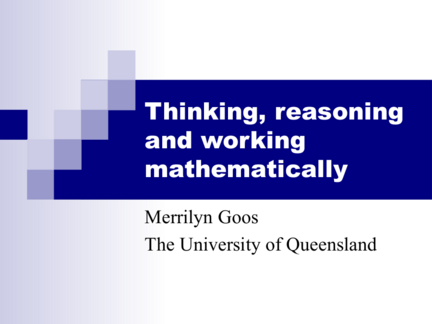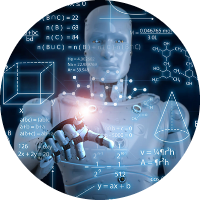# Thinking Reasoning and Working MathematicallyContributed by:The basic outline of this presentation is:

1. What is mathematical thinking?
2. What teaching approaches can develop students’ mathematical thinking?
3. How does the syllabus support current research on mathematical thinking?
4. How can we engage students in thinking, reasoning, and working mathematically?
1. Thinking, reasoning
and working
Merrilyn Goos
The University of Queensland
2. Why is mathematics important?
 Mathematics is used in daily living, in civic life,
and at work (National Statement)
 Mathematics helps students develop attributes of a
lifelong learner (Qld Years 1-10 Mathematics
Syllabus)
3.  What is mathematical thinking?
 What teaching approaches can develop students’
mathematical thinking?
 How does the syllabus support current research on
mathematical thinking?
 How can we engage students in thinking,
reasoning and working mathematically?
4. What is “mathematical thinking”?
5. Some mathematical thinking …
 How far is it around the moon?
 How many cars does this represent?
 How long would it take to advertise this number
of cars?
6. How far is it around the moon?
diameter = 3445km
circumference = π  3445km
= 10,822km
7. How many cars?
Number of cars
= 10,822  1000  (average length of one car in metres)
= 2.7 million cars
= (2.7  106 cars) 
(2.7  103 cars per week)
= 1000 weeks
= 19.2 years
9. What is “mathematical thinking?”
Cognitive
processes
knowledge strategies
skills
10. What is “mathematical thinking?”
Metacognitive regulation
awareness
processes
Cognitive
processes
knowledge strategies
skills
11. What is “mathematical thinking?”
beliefs affects
Dispositions
Metacognitive regulation
awareness
processes
Cognitive
processes
knowledge strategies
skills
12. Mathematical thinking means …
mathematical
point of view
13. How do you know when you understand
something in mathematics?
14. How do you know when you understand
something in mathematics?
Category Frequency Proportion
II Affective response 35 0.11
III Makes sense 52 0.16
IV Application/transfer 27 0.08
V Explain to others 24 0.07
15. Mathematical understanding involves …
 knowing-that (stating)
 knowing-how (doing)
 knowing-why (explaining)
 knowing-when (applying)
Understanding means making connections
between ideas, facts and procedures.
16. What teaching approaches can
develop mathematical thinking?
Develop a mathematical “point of view”
Knowing that, how, why, when
Making connections within and
beyond mathematics
Investigative approach
17. Calculators in Primary Mathematics
 6 Melbourne schools:
1000 children & 80 teachers
 Prep-Year 4
 Children given their own
arithmetic calculators
 Teachers not provided with
activities or program
18. Calculators in Primary Mathematics
 How can calculators be used in lower primary
mathematics classrooms?
 What effects will the calculators have on teachers’
beliefs, classroom practice, and expectations of
children?
 What effects will the calculators have on
children’s learning of number concepts?
19. How were calculators used?
Exploring number concepts:
10 + 10 = = = =
Alex (5 yrs): I’m counting by tens and I’m up to 300!
Teacher: And what would you like to get to?
Alex: A thousand and fifty!
20. How were calculators used?
Exploring number concepts: Counting
9 + 9 = = = 9
18
27
Counting by 9s and
36
recording the output 45
on a number roll 54
63
72
81
21. How were calculators used?
Exploring number concepts: Counting backwards
Underground numbers!
22. How were calculators used?
Exploring number concepts: Place value
9345 “Nine thousand three hundred and forty-five”
6056 “Six thousand and fifty-six”
9000000000 “Nine billion!”
23. What were the effects on teachers?
 More open-ended teaching practices
“I’m not so worried about them finding out things they
won’t understand any more … I think I’m being a lot more
open-ended with their activities.”
 More discussion and sharing of children’s ideas
“It certainly encouraged me to talk to the children much
more and discuss how did they do this, why did they do that,
and getting them to justify what they’re doing.”
24. What were the effects on children’s
number learning?
 Interviews and written tests with project children and
control group in Years 3 and 4.
 Two types of test:
(1) paper & pencil
(2) calculator.
 Two types of interview:
(1) choose any calculation method or device
(2) mental computation only
 Project children had better overall performance.
25. Open and closed mathematics
Amber Hill School
 Textbooks
 Short, closed questions
 Teacher exposition every day
 Individual work
 Disciplined
26. Open and closed mathematics
Amber Hill School Phoenix Park School
 Textbooks  Projects
 Short, closed questions  Open problems
 Teacher exposition every day  Teacher exposition rare
 Individual work  Group discussions
 Disciplined  Relaxed
27. Open and closed mathematics
 How do students view the world of the school
mathematics classroom?
 How do their views impact on the mathematical
knowledge they develop and their ability to use
this knowledge?
28. What were students’ views about school
Amber Hill: monotony and meaninglessness
“I wish we had different questions, not
three pages of sums on the same thing.”
“In maths there’s a certain formula to
get from A to B, and there’s no other
way to get to it.”
“In maths you have to remember; in
other subjects you can think about it.”
29. What were students’ views about school
Phoenix Park: thinking and connections
“It’s more the thinking side to sort of look
at everything you’ve got and think about
how to solve it.”
“Here you have to explain how you got [the
“When I’m out of school now, I can connect
back to what I done in class so I know what
I’m doing.”
30. What mathematical knowledge did the
students develop?
% of Students
Amber Hill Phoenix Park
GCSE: pass 71% 88%
knowing-that knowing-why
knowing-how knowing-when
31. How does the syllabus support current
research on mathematical thinking?
 Syllabus rationale: what is mathematics?
 Syllabus organisation: three levels of outcomes
 Planning with outcomes: using investigations,
making connections
32. Years 1-10 syllabus Rationale
Mathematics is a unique and
powerful way of viewing the
world to investigate patterns,
order, generality and uncertainty.
33. Years 1-10 syllabus organisation
Attributes of a life long learner
Key Learning Area outcomes
Core and discretionary
learning outcomes
34. Attributes of a lifelong learner
A lifelong learner is:
 A knowledgeable person with deep understanding
 A complex thinker
 A responsive creator
 An active investigator
 An effective communicator
 A participant in an interdependent world
 A reflective and self-directed learner
35. Years 1-10 syllabus organisation
Attributes of a life long learner
Key Learning Area outcomes
Core and discretionary
learning outcomes
36. Mathematics KLA Outcomes
(thinking, reasoning and working mathematically)
 Understand the nature of mathematics as a dynamic human
endeavour …
 Interpret and apply properties and relationships …
 Identify and analyse information …
 Create mathematical models …
 Pose and solve mathematical problems …
 Use the concise language of mathematics …
 Collaborate and cooperate, challenge the reasoning of others …
 Reflect on, evaluate and apply their mathematical learning …
37. Years 1-10 syllabus organisation
Attributes of a life long learner
Key Learning Area outcomes
Core and discretionary
learning outcomes
38. Core Learning Outcomes
Levels
Strands 1 2 3 4 5 6
Patterns &
Chance & data
39. Planning with outcomes:
Making connections
When planning units of work, teachers could
combine learning outcomes from:
 within a strand of a KLA
 across strands within a KLA
 across levels within a KLA
 across KLAs
40. Planning with outcomes:
An investigative approach
The focus for planning within and across key
learning areas can be framed in terms of:
 a problem to be solved
 a question to be answered
 a significant task to be completed
 an issue to be explored
41. How can we engage students in thinking,
reasoning and working mathematically?
An investigation that combines outcomes:
 within a strand of a KLA
 across strands within a KLA
 across levels within a KLA
Pyramids of
 across KLAs Egypt investigation
42. Investigations across KLAs:
The curriculum integration project
 The impact of the mediaeval plagues
 The mystery of the Mayans
 Managing the Bulimba Creek catchment
 Building the pyramids of Egypt
43. Pyramids of Egypt Investigation
You have been declared
Pharaoh of Egypt!
As a monument to your
reign, you decide to build a
Prepare a feasibility study
for the construction project,
including a scale model of
44. Pyramids of Egypt investigation
SOSE/History Content Mathematics Content
 When were the pyramids  Measurement of time,
built? (dating methods) length, mass, area, volume
 Political/social structure of  Data presentation and
ancient Egypt interpretation
 Geography of Egypt  Ratio and proportion (scale)
 Religious/burial practices  Angles, 2D and 3D shapes
 Pyramid construction
methods
45. How big are the pyramids?
If Khafre’s pyramid were as tall as this room,
how tall would you be?
46. How were the pyramids built?
Volume of Khufu’s pyramid = 2,583,283m3
 If the density of limestone is 2280 kg/m3, what is the total
weight of Khufu’s pyramid?
Weight of pyramid = 5,889,886 tons
 If the average weight of a limestone block is 2.5 tons, how
many blocks comprise Khufu’s pyramid?
Number of blocks = 2,355,954
 Khufu reigned for 23 years. How many blocks of limestone
needed to be delivered to the pyramid every hour for it to be
completed within his reign?
12 blocks/hr all year or 35 blocks/hr during inundation period
47. Pyramids of Egypt investigation
SOSE syllabus strand Mathematics syllabus strands
 Time, continuity and  Measurement
change  Chance and Data
 Number
 Space
48. Thinking, reasoning
and working
Merrilyn Goos
The University of Queensland